### Home > CALC > Chapter 6 > Lesson 6.3.1 > Problem6-86

6-86.
1. Sketch the graph of y = 2x and its inverse. Label all intercepts. Homework Help ✎

1. Find the equation of the line tangent to the graph of y = 2x at its y-intercept.

2. Find the equation of the line tangent to the graph of y = log2 x at its x-intercept.

3. Graph both of these lines. What is their relationship?

4. Use the tangent line in part (a) to approximate the value of y when x = 0.1. Is your estimate an under or over approximation of the actual value? How do you know using concavity?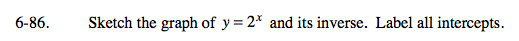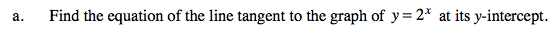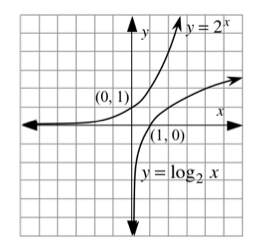Use point-slope form.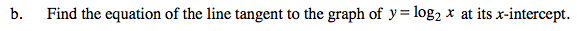Refer to hint in part (a).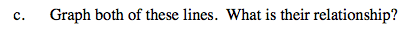Sketch the tangent lines. Are they parallel? Are they perpendicular? Neither?
Look at their equations... describe a pattern you see in their slopes.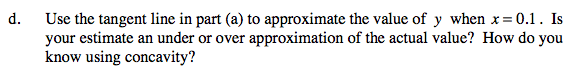Recall that the concavity of the graph determines whether tangent lines lie above or below the curve.